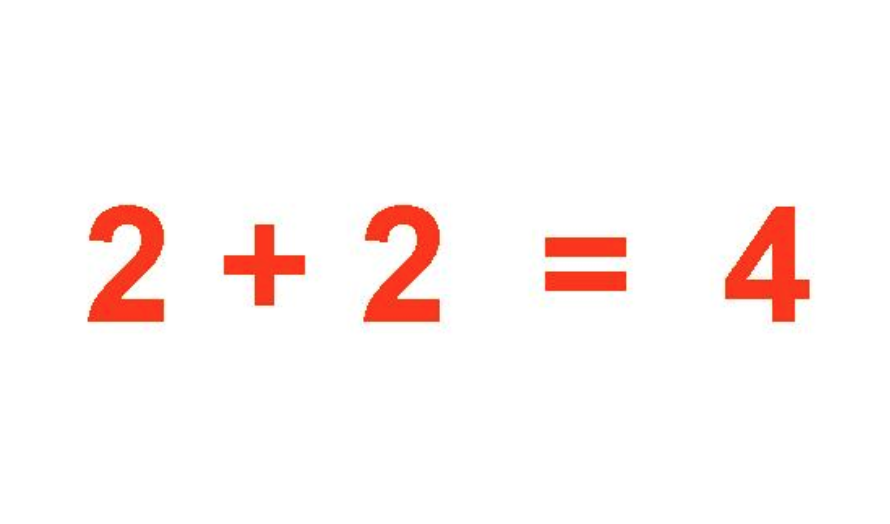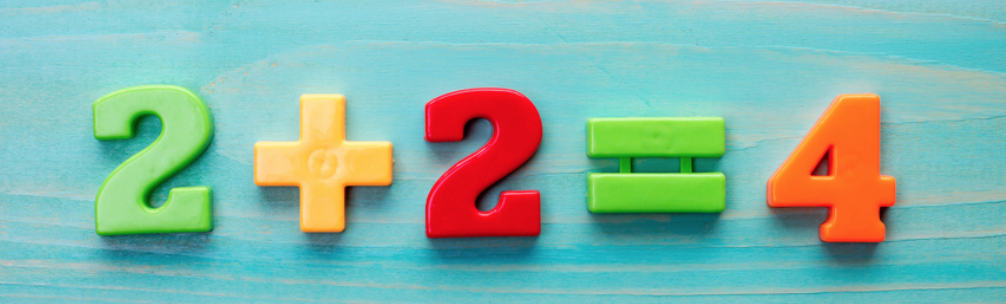# Thoughts about Truth (Deep Think #2)

Thoughts about Truth (Deep Think #2)When I say “thoughts about Truth,” I am talking about “capital T” Truth, which for me is a synonym for God. God is Truth. Truth is God. God, and therefore Truth, is infinite, all-powerful, ever-present and good. God (Truth) is supreme, imperative, inalterable, fact.

We reflect God as Truth; the first chapter of Genesis claims we are made in God’s “image and likeness.” This doesn’t just mean we are honest, or we naturally all abhor fake news. We can go much deeper with this idea. Truth is a rock we can stand on, and we are benefited by turning to that when things look squishy. I return to God as Truth over and over again because it feels so solid and unchangeable, reliable and immovable.

Like Jesus using parables that are easy to grasp, I find it helpful to look at a simple fact, and extrapolate from there, to better understand what is actually going on under any circumstance.  I often use the example of 2+2=4 as an idea of Truth to explain this to my clients.

Each one of us is a complete and perfect idea of God (Truth), but sometimes it is hard to see ourselves that way. However, it is not so hard to see 2+2=4 that way. So when we can see the Truth in 2+2=4, we can start to see those same aspects in ourselves.

For example:

2+2=4 is never unhappy.

2+2=4 is never afraid.

2+2=4 is never exhausted.

2+2=4 is never unsettled.

2+2=4 never disobeys a commandment or law.

2+2=4 is never sick.

2+2=4 is never wrong.

2+2=4 is never unkind.

2+2=4 is never unsure or insecure.

2+2=4 is never vulnerable.

2+2=4 is never out of control.

2+2=4 never gets broken.

2+2=4 is never volatile.

2+2=4 is never threatened or thwarted.

2+2=4 is never inflated or egotistical.

2+2=4 is never ungrateful.

2+2=4 is never impatient.

2+2=4 is not material or physical.

2+2=4 is not sad or lonely.

2+2=4 is not in pain.

2+2=4 is not competitive.

2+2=4 is not too fat or too thin.

2+2=4 is not jealous or envious.

2+2=4 is not defined by race, gender, or nationality.

2+2=4 was never a victim.

2+2=4 was never hurt.

2+2=4 was never left out.

2+2=4 doesn’t have a mind of its own.

2+2=4 doesn’t get frustrated or aggravated.

2+2=4 doesn’t make mistakes.

2+2=4 doesn’t have feelings or emotions.

2+2=4 has no personality.

2+2=4 has no opinion.

2+2=4 does have an impact and effect.

2+2=4 does have a specific, unique, and very vital job to do.

2+2=4 always has everything it needs.

2+2=4 is always appreciated.

2+2=4 is always confident.

2+2=4 is always truthful.

2+2=4 is always rested and alert.

2+2=4 is always prepared and ready.

2+2=4 always gets along harmoniously.

2+2=4 is always safe.

2+2=4 is always unselfish.

2+2=4 is always spiritual.

2+2=4 is always calm.

2+2=4 is always satisfied.

2+2=4 is universally loved.

2+2=4 is perpetually utilized.

2+2=4 is essential, necessary, important.

2+2=4 is just, equitable, impartial.

2+2=4 is valuable.

2+2=4 is relied upon.

2+2=4 is persuasive.

2+2=4 is indelibly strong.

2+2=4 is objective.

2+2=4 intentional.

2+2=4 is impersonal.

2+2=4 is always at peace.

2+2=4 is present everywhere.

2+2=4 is complete.

2+2=4 serves and reflects Truth.

2+2=4 is an idea of God. So are you in very similar ways.  Ultimately, you are not a material mind or body. You are not flawed, scared, emotive, deprived, or failing.

As Truth’s image and likeness, you have dominion. Exercise and claim that and see what a difference it makes. What is true for one idea of God is true for another. Consider 2+2=4 and then consider what that means for yourself. Make adjustments at the places during that list above where your thought lurched forward with an aha.

The only difference between 2+2=4 and you is that you are God’s best idea. Truth takes care of 2+2=4 very dependably. How much more cherished and fulfilled and secure are you?I work to amplify good wherever I find it. I love color, texture, beauty, great ideas, nature, metaphor, deliciousness, genuine spirituality, and exploring new territory. I encourage authenticity, nurture creativity, champion sustainability, promote peace, and hope to foster a new renaissance where we all are free to be our most fulfilled, multifaceted, and terrific selves. Read more here.

### 1 Comment

1.Gillian 5 years ago

Thank you so much Polly… 2+2=4 has really made me sit up and take notice.

And thank you for all the inspiration you share so generously – I am deeply grateful – HNY X

Send this to friend